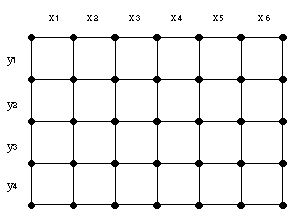## 2012年11月1日 星期四

### [離散] Euler Circuit and Hamiltonian Cycle Prove

This is my note for learning and proving Euler Circuit and Hamiltonian Cycle problems.

Problem 1: Given a 2-dimension mesh graph of m rows and n columns as follows (m>2, n>2)Courtesy of http://www.aya.or.jp/~babalabo/Hamilton/Draft1-2.html
(a) Please show that if both m and n are even number, the mesh graph doesn't contain an Euler Circuit.
(b) Please show that if both m and n are even number, the mesh graph doesn't contain an Euler Trail.
(c) Please show that if both m and n are even number, the mesh graph contains a Hamiltonian Cycle.
(d) Please show that if both m and n are odd number, the mesh graph contains a Hamiltonian Path.

Proof :

(a) Since the vertices at four angles of the mesh graph must have degree value in 3.
By the theorem, if a graph has Euler Circuit, then the degree for all vertex must be even.
Hence the graph does not contain an Euler Circuit.

(b) By the theorem, if a graph has Euler Path, then it must contain only 0 or 2 vertices have odd degree.
As the result of (a), there are at least 4 vertices have degree value in 3.
Hence the graph does not contain an Euler Trail.

(c) Since m is even, then the mesh graph is a bipartite graph.
1  2  1  2  ...
2  1  2  1  ...
1  2  1  2  ...
2  1  2  1  ...
1  2  1  2  ...
:   :   :   :   :
1  2  1  2  ...
2  1  2  1  ...
And the number of 1 and 2 are same of each column, that is |V1| = |V2| = m/2
By the theorem, if |V1| = |V2|, then the bipartite contains Hamiltonian Cycle.
Hence, the mesh graph contains a Hamiltonian Cycle.

(d) When m and n are odd value, the value of |V1| and |V2| is differentiate in 1.
Such that the absolute value of |V1| - |V2| <= 1.
By the theorem, if the absolute value of |V1| - |V2| <= 1, then the bipartite contains Hamiltonian Path.
So that the bipartite mesh graph contains a Hamiltonian Path.
----------------------------------------------------------------------------------------------------------------------
Problem 2 : Prove that a connected graph with a bridge dose not have a Hamiltonian cycle.

Proof :
Take out the bridge of G will result in two components G1 and G2.
By contradiction, suppose that G has Hamiltonian cycle, then we can arbitrarily choose one vertex in G and traverse all the other vertices from it without passing any edge twice and finally get back to the start vertex. When the traversal visits via the bridge from G1 to G2, we must can find another edge to get back to G1 from G2, that is, there must exist another edge connecting G1 and G2 except bridge. It's contrary with the definition of bridge. Hence, G does not have Hamiltonian cycle.
----------------------------------------------------------------------------------------------------------------------
Problem 3: Show that a connected graph G with 11 vertices and 53 edges must have Hamiltonian Cycle but no Euler Circuit.

Proof :
2|E| = 53*2 = 106
The maximum value of vertices is C(11,2) = 55
When the value of vertices is 55, deg(v) =  n - 1 = 11-1 = 10 for all vertex.
But there are 53 edges, this implies that the graph is taken two edges out of the complete graph with 11 vertices.

(1) Case I : At least 3 vertices degree < 10
Suppose take 2 edges out of 1 vertex, its degree becomes 8.
Then take out the edge of the two vertices which are connecting to the former vertex, both of their degree become 9.
Since there exist vertices with odd degree.
Hence, G does not have Euler Circuit.
For any 2 vertices x, y, x!=y and are not adjacent, deg(x) + deg(y) = sum of two of {8,9,10} > 11.
Hence, the Hamiltonian Cycle exist.

(2) Case II: At most 4 vertices degree < 10
Suppose the two taken out edges are belong two different pair of vertices, {a, b} and {c, d}, respectively.
Then deg(a) = deg(b) = deg(c) = deg(d) = 9
Since there exist vertices with odd degree.
Hence, G does not have Euler Circuit.
For x, y, x!=y and are not adjacent, deg(x) + deg(y) = sum of two of {9, 10} > 11.
Hence, the Hamiltonian Cycle exist.

#### 1 則留言:

1.第三題中文筆記:

11vertices
53edges

53 * 2 = 106

vertices 最多是 C(11,2) = 55
當vertices為最大值時，每個點的deg(v) = n - 1 = 11-1 = 10
但是只有 53 個邊 (表示拿掉兩個邊)

至少有3個點 deg(v) < 10
這個情況:
假設1個點拿掉2邊(deg = 8)，另2個點各拿掉與前面那個點相連之邊(deg 各為 9) ，
因存在奇數degree的點，則EC不存在
又任意兩個點 x, y, x!=y且不相鄰, deg(x) + deg(y) = sum of two of {8,9,10} > 11，所以存在 HC

最多4個點 deg < 10
這個情況:
假設拿掉的兩個邊各屬不同兩對點{a,b}和{c,d}
則 deg(a,b,c,d) 都 = 9
因為存在奇數degree的點，所以不存在EC
又任意兩個點 x, y, x!=y 且不相鄰, deg(x) + deg(y) = sum of two of {9, 10} > 11 所以存在 HC